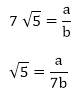# RD Sharma Class 10 Ex 1.5 Solutions Chapter 1 Real Numbers

In this chapter, we provide RD Sharma Class 10 Ex 1.5 Solutions Chapter 1 Real Numbers for English medium students, Which will very helpful for every student in their exams. Students can download the latest RD Sharma Class 10 Ex 1.5 Solutions Chapter 1 Real Numbers pdf, Now you will get step by step solution to each question.

# Chapter 1: Real Numbers Exercise – 1.5

### Question: 1

Show that the following numbers are irrational.

(i) 7 √5

(ii) 6 + √2

(iii) 3 – √5

### Solution:

(i) Let us assume that 7 √5 is rational. Then, there exist positive co primes a and b such that

###We know that √5 is an irrational number

Here we see that √5 is a rational number which is a contradiction.

(ii) Let us assume that 6+√2 is rational. Then, there exist positive co primes a and b such that

Here we see that √2 is a rational number which is a contradiction as we know that √2 is an irrational number

Hence 6 + √2 is an irrational number

(iii) Let us assume that 3 – √5 is rational. Then, there exist positive co primes a and b such that

Here we see that √5 is a rational number which is a contradiction as we know that √5 is an irrational number

Hence 3 – √5 is an irrational number.

### Question: 2

Prove that the following numbers are irrationals.

(i) 2√7

(ii) 3/(2√5)

(iii) 4 + √2

(iv)  5√2

### Solution:

(i) Let us assume that 2√7 is rational. Then, there exist positive co primes a and b such that

√7 is rational number which is a contradiction

Hence 2√7 is an irrational number

(ii) Let us assume that 3/(2√5) is rational. Then, there exist positive co primes a and b such that

√5 is rational number which is a contradiction

Hence 3/(2√5) is irrational.

(iii) Let us assume that 3/(2√5) is rational. Then, there exist positive co primes a and b such that

√2 is rational number which is a contradiction

Hence 4+ √2 is irrational.

(iv) Let us assume that 5√2 is rational. Then, there exist positive co primes a and b such that

√2 is rational number which is a contradiction

Hence 5√2 is irrational

### Question: 3

Show that 2 – √3 is an irrational number.

### Solution:

Let us assume that 2 – √3 is rational. Then, there exist positive co primes a and b such that

Here we see that √3 is a rational number which is a contradiction

Hence 2- √3 is irrational

### Question: 4

Show that 3 + √2 is an irrational number.

### Solution:

Let us assume that 3 + √2 is rational. Then, there exist positive co primes a and b such that

Here we see that √2 is a irrational number which is a contradiction

Hence 3 + √2 is irrational

### Question: 5

Prove that 4 – 5√2 is an irrational number.

### Solution:

Let us assume that 4 – 5√2 is rational. Then, there exist positive co primes a and b such that

This contradicts the fact that √2 is an irrational number

Hence 4 – 5√2 is irrational

### Question: 6

Show that 5 – 2√3 is an irrational number.

### Solution:

Let us assume that 5 -2√3 is rational. Then, there exist positive co primes a and b such that

This contradicts the fact that √3 is an irrational number

Hence 5 – 2√3 is irrational

### Question: 7

Prove that 2√3 – 1 is an irrational number.

### Solution:

Let us assume that 2√3 – 1 is rational. Then, there exist positive co primes a and b such that

This contradicts the fact that √3 is an irrational number

Hence 5 – 2√3 is irrational

### Question: 8

Prove that 2 – 3√5 is an irrational number.

### Solution:

Let us assume that 2 – 3√5 is rational. Then, there exist positive co primes a and b such that

This contradicts the fact that √5 is an irrational number

Hence 2 – 3√5 is irrational

### Question: 9

Prove that √5 + √3 is irrational.

### Solution:

Let us assume that √5 + √3 is rational. Then, there exist positive co primes a and b such that

Here we see that √3 is a rational number which is a contradiction as we know that √3 is an irrational number

Hence √5 + √3 is an irrational number

### Question: 10

Prove that √3 + √4 is irrational.

### Solution:

Let us assume that √3 + √4 is rational. Then, there exist positive co primes a and b such that

Here we see that √3 is a rational number which is a contradiction as we know that √3 is an irrational number

Hence √3 + √4   is an irrational number

### Question: 11

Prove that for any prime positive integer p, √p is an irrational number.

### Solution:

Let us assume that √p is rational. Then, there exist positive co primes a and b such that

⇒ pb2 = a2

⇒ p|a2 ⇒ p|a2

⇒ p|a ⇒ p|a

⇒ a = pc for some positive integer c ⇒ a = pc for some positive integer c

b2p = a2

⇒ b2p = p2c2 ( ∵ a = pc)

⇒ p|b(since p|c2p) ⇒ p|b2(since p|c2p)

⇒ p|b ⇒ p|a and p|b

This contradicts the fact that a and b are co primes

Hence √p is irrational

### Question: 12

If p, q are prime positive integers, prove that √p + √q is an irrational number.

### Solution:

Let us assume that √p + √q is rational. Then, there exist positive co primes a and b such that

Here we see that √q is a rational number which is a contradiction as we know that √q is an irrational number

Hence √p + √q  is an irrational number

All Chapter RD Sharma Solutions For Class10 Maths

I think you got complete solutions for this chapter. If You have any queries regarding this chapter, please comment on the below section our subject teacher will answer you. We tried our best to give complete solutions so you got good marks in your exam.